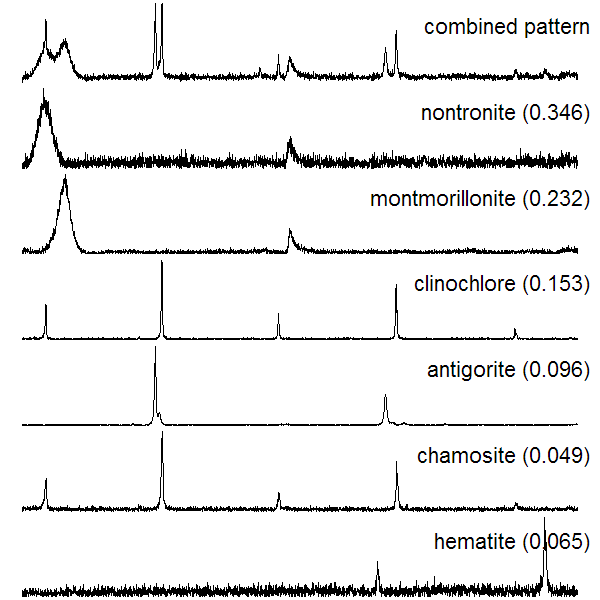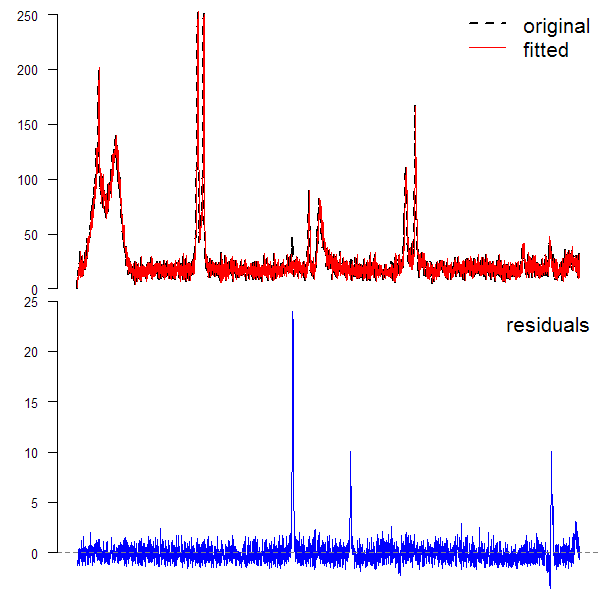f.noise {aqp} R Documentation

## Example Objective Function for Full-Pattern Matching

### Description

Basic objective function that can be used as a starting point for developing XRD full-pattern matching strategies. [details pending...]

### Usage

```f.noise(inits, pure.patterns, sample.pattern, eps.total = 0.05)
```

### Arguments

 `inits` vector of initial guesses for mineral fractions, last item is a noise component `pure.patterns` a matrix of XRD patterns of pure samples, resampled to the same twotheta resolution and rescaled according to an external standard `sample.pattern` the unkown or composite pattern, aligned to the same twotheta axis as the pure patterns and rescaled to an external standard `eps.total` precision of comparisons; currently not used

### Details

This is similar to the work of Chipera and Bish (2002), using the methods described in (Bish, 1994). If the flexibility of a custom objective function is not required, the linear model framework should be sufficient for pattern fitting. GLS should be used if realistic standard errors are needed.

### Value

the sum of absolute differences between the unkown pattern and combination of pure patterns for the current set of mixture proportions

### Author(s)

Dylan E. Beaudette

### References

Chipera, S.J., & Bish, D.L. (2002) FULLPAT: A full-pattern quantitative analysis program for X-ray powder diffraction using measured and calculated patterns. J. Applied Crystallography, 35, 744-749.

Bish, D. 1994. Quantitative Methods in Soil Mineralogy, in Quantitative X-Ray Diffraction Analysis of Soil. Amonette, J. & Zelazny, L. (ed.) Soil Science Society of America, pp 267-295.

`resample.twotheta`

### Examples

``````# sample data
data(rruff.sample)

# get number of measurements
n <- nrow(rruff.sample)

# number of components
n.components <- 6

# mineral fractions, normally we don't know these
w <- c(0.346, 0.232, 0.153, 0.096, 0.049, 0.065)

# make synthetic combined pattern
# scale the pure substances by the known proportions
rruff.sample\$synthetic_pat <- apply(sweep(rruff.sample[,2:7], 2, w, '*'), 1, sum)

# add 1 more substance that will be unknown to the fitting process
rruff.sample\$synthetic_pat <- rruff.sample\$synthetic_pat +
(1 - sum(w)) * rruff.sample[,8]

# try adding some nasty noise
# rruff.sample\$synthetic_pat <- apply(sweep(rruff.sample[,2:7], 2, w, '*'), 1, sum) +
# runif(n, min=0, max=100)

# look at components and combined pattern
par(mfcol=c(7,1), mar=c(0,0,0,0))
plot(1:n, rruff.sample\$synthetic_pat, type='l', axes=FALSE)
legend('topright', bty='n', legend='combined pattern', cex=2)
for(i in 2:7)
{
plot(1:n, rruff.sample[, i], type='l', axes=FALSE)
legend('topright', bty='n',
legend=paste(names(rruff.sample)[i], ' (', w[i-1], ')', sep=''), cex=2)
}
````````````## fit pattern mixtures with a linear model
l <- lm(synthetic_pat ~ nontronite + montmorillonite + clinochlore
+ antigorite + chamosite + hematite, data=rruff.sample)

summary(l)
``````
``````##
## Call:
## lm(formula = synthetic_pat ~ nontronite + montmorillonite + clinochlore +
##     antigorite + chamosite + hematite, data = rruff.sample)
##
## Residuals:
##     Min      1Q  Median      3Q     Max
## -3.5972 -0.6693 -0.1533  0.4238 24.0017
##
## Coefficients:
##                  Estimate Std. Error t value Pr(>|t|)
## (Intercept)     1.2348183  0.0509941   24.21   <2e-16 ***
## nontronite      0.3449640  0.0008044  428.83   <2e-16 ***
## montmorillonite 0.2314518  0.0004195  551.68   <2e-16 ***
## clinochlore     0.1520822  0.0007385  205.92   <2e-16 ***
## antigorite      0.0959113  0.0002042  469.70   <2e-16 ***
## chamosite       0.0498834  0.0013887   35.92   <2e-16 ***
## hematite        0.0746220  0.0010858   68.72   <2e-16 ***
## ---
## Signif. codes:  0 '***' 0.001 '**' 0.01 '*' 0.05 '.' 0.1 ' ' 1
##
## Residual standard error: 1.475 on 2993 degrees of freedom
## Multiple R-squared:  0.9971, Adjusted R-squared:  0.9971
## F-statistic: 1.715e+05 on 6 and 2993 DF,  p-value: < 2.2e-16
``````
``````par(mfcol=c(2,1), mar=c(0,3,0,0))
plot(1:n, rruff.sample\$synthetic_pat, type='l', lwd=2, lty=2, axes=FALSE,
xlab='', ylab='')
lines(1:n, predict(l), col=2)
axis(2, cex.axis=0.75, las=2)
legend('topright', legend=c('original','fitted'), col=c(1,2), lty=c(2,1),
lwd=c(2,1), bty='n', cex=1.25)

plot(1:n, resid(l), type='l', axes=FALSE, xlab='', ylab='', col='blue')
abline(h=0, col=grey(0.5), lty=2)
axis(2, cex.axis=0.75, las=2)
legend('topright', legend=c('residuals'), bty='n', cex=1.25)
````````````## fitting by minimizing an objective function (not run)

# SANN is a slower algorithm, sometimes gives strange results
# default Nelder-Mead is most robust
# CG is fastest --> 2.5 minutes max
# component proportions (fractions), and noise component (intensity units)
# initial guesses may affect the stability / time of the fit

## this takes a while to run
# # synthetic pattern
# o <- optim(par=c(0.1, 0.1, 0.1, 0.1, 0.1, 0.1, 0.1), f.noise,
# method='CG', pure.patterns=rruff.sample[,2:7],
# sample.pattern=rruff.sample\$synthetic_pat)
#
#
# # estimated mixture proportions
# o\$par
#
# # compare with starting proportions
# rbind(o\$par[1:n.components], w)
#
# # if we had an unkown pattern we were trying to match, compare fitted here
# # compute R value 0.1 - 0.2 considered good
# # sum(D^2) / sum(s)
# # o\$value / sum(rruff.sample\$sample)
#
# # plot estimated mixture vs sample
# # combine pure substances
# pure.mixture <- apply(sweep(rruff.sample[, 2:7], 2, o\$par[1:n.components], '*'), 1, sum)
#
# # add in noise
# noise.component <- o\$par[n.components+1]
# est.pattern <- pure.mixture + noise.component
#
#
# # plot results
# par(mfcol=c(2,1), mar=c(0,3,0,0))
# plot(1:n, rruff.sample\$synthetic_pat, type='l', lwd=2, lty=2, axes=FALSE,
# xlab='', ylab='')
# lines(1:n, est.pattern, col=2)
# lines(1:n, rep(noise.component, n), col=3)
# axis(2, cex.axis=0.75, las=2)
# legend('topright', legend=c('original','fitted','noise'), col=c(1,2,3), lty=c(2,1,1),
# lwd=c(2,1,1), bty='n', cex=1.25)
#
# plot(1:n, rruff.sample\$synthetic_pat - est.pattern, type='l', axes=FALSE,
# xlab='', ylab='')
# abline(h=0, col=grey(0.5), lty=2)
# axis(2, cex.axis=0.75, las=2)
# legend('topright', legend=c('difference'), bty='n', cex=1.25)
#
``````

[Package aqp version 1.9.1 Index]